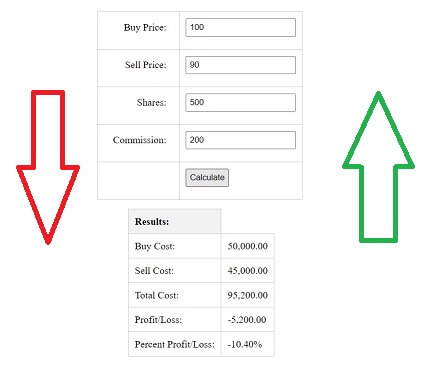# PHP Script for Stock Profit Loss Calculator with Commission Input [Source Code]

Calculate your stock profit or loss with this PHP script. This calculator includes a commission input, allowing you to accurately calculate your total costs. Simply enter your buy and sell prices, as well as the number of shares, to get started. Results are displayed in a clear, organized table. Keep track of your investments and make informed decisions with this powerful tool.

Check the Live Demo of the script here## CSS Code to Style Form

```<style>
table {
border-collapse: collapse;
margin: 20px auto;
}
table td, table th {
border: 1px solid #ccc;
}
table th {
background-color: #f2f2f2;
text-align: left;
}
input[type="number"], input[type="submit"] {
margin-bottom: 10px;
}
label {
display: inline-block;
width: 100px;
text-align: right;
margin-bottom: 10px;
}
</style>
```

## PHP Code to Calculate Profit and Loss

```<?php
if(\$_SERVER['REQUEST_METHOD'] == 'POST') {
\$sell_price = floatval(\$_POST['sell_price']);
\$shares = intval(\$_POST['shares']);
\$commission = floatval(\$_POST['commission']);

\$sell_cost = \$sell_price * \$shares;
\$total_cost = \$buy_cost + \$sell_cost + \$commission;

\$profit_loss = \$sell_cost - \$buy_cost - \$commission;
\$percent_profit_loss = (\$profit_loss / \$buy_cost) * 100;
}
?>
```

## HTML and PHP Code For Input and Display Results

```<form method="post">
<table>
<tr>
</tr>
<tr>
<td><label>Sell Price:</label></td>
<td><input type="number" step="any" name="sell_price" required></td>
</tr>
<tr>
<td><label>Shares:</label></td>
<td><input type="number" name="shares" required></td>
</tr>
<tr>
<td><label>Commission:</label></td>
<td><input type="number" step="any" name="commission" value="0"></td>
</tr>
<tr>
<td></td>
<td><input type="submit" value="Calculate"></td>
</tr>
</table>
</form>

<?php if(\$_SERVER['REQUEST_METHOD'] == 'POST') { ?>
<table>
<tr>
<th>Results:</th>
</tr>
<tr>
</tr>
<tr>
<td>Sell Cost:</td>
<td><?= number_format(\$sell_cost, 2) ?></td>
</tr>
<tr>
<td>Total Cost:</td>
<td><?= number_format(\$total_cost, 2) ?></td>
</tr>
<tr>
<td>Profit/Loss:</td>
<td><?= number_format(\$profit_loss, 2) ?></td>
</tr>
<tr>
<td>Percent Profit/Loss:</td>
<td><?= number_format(\$percent_profit_loss, 2) ?>%</td>
</tr>
</table>
<?php } ?>
```

you can also check GitHub for full source code

•Article By :